Examples

Chapter 3 Class 10 Pair of Linear Equations in Two Variables
Serial order wise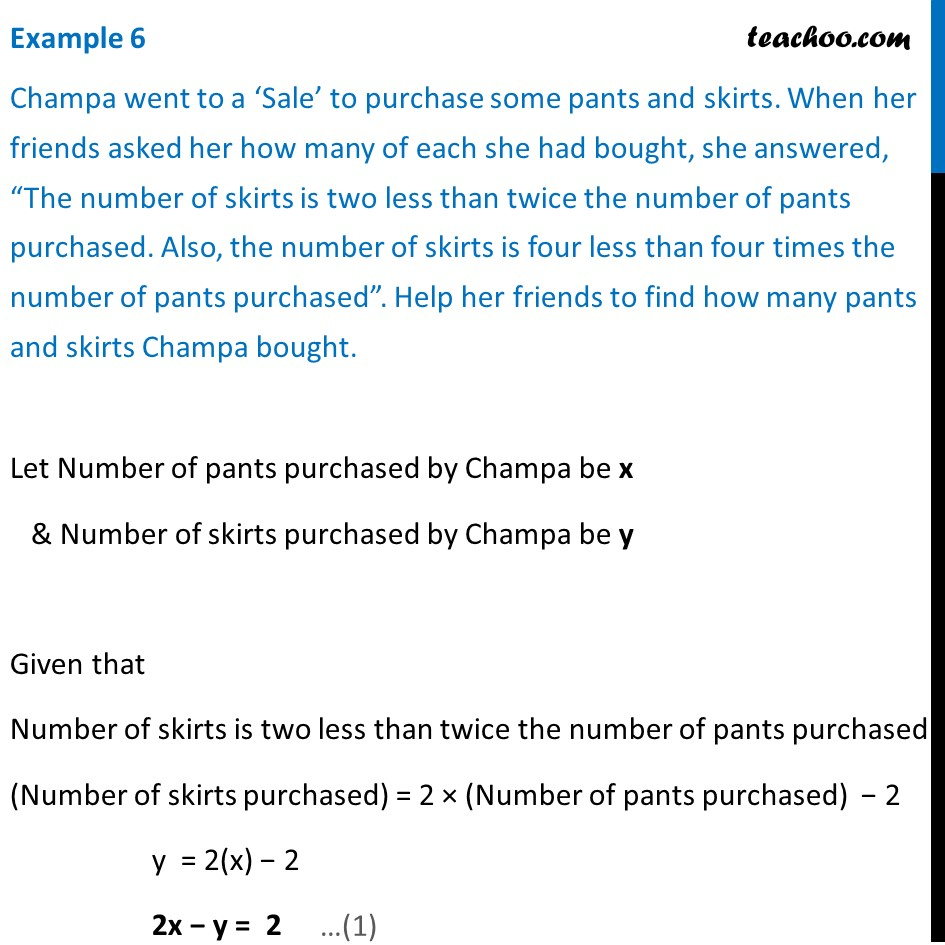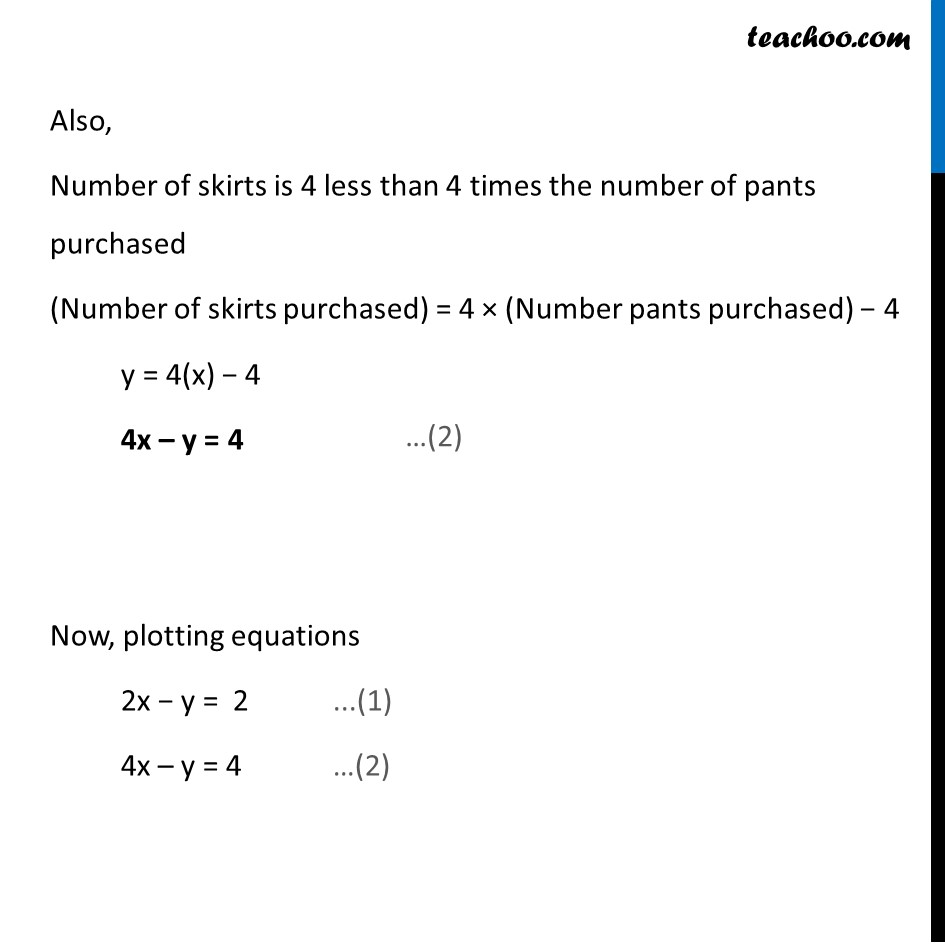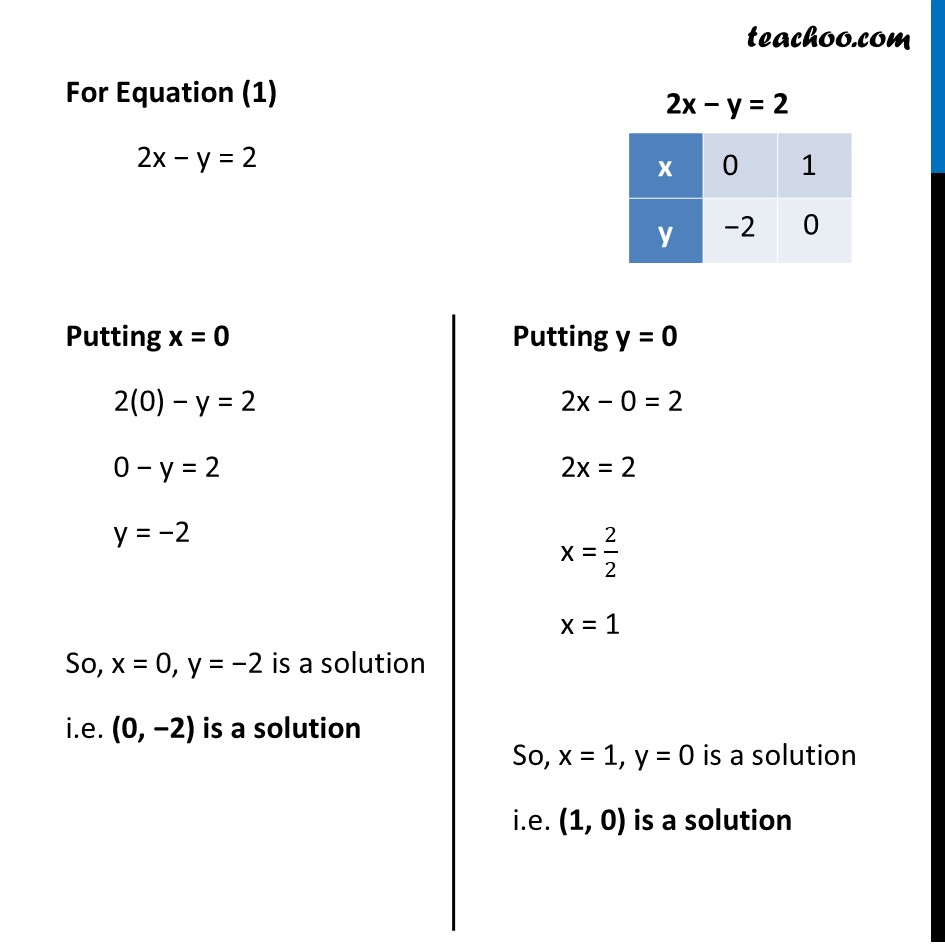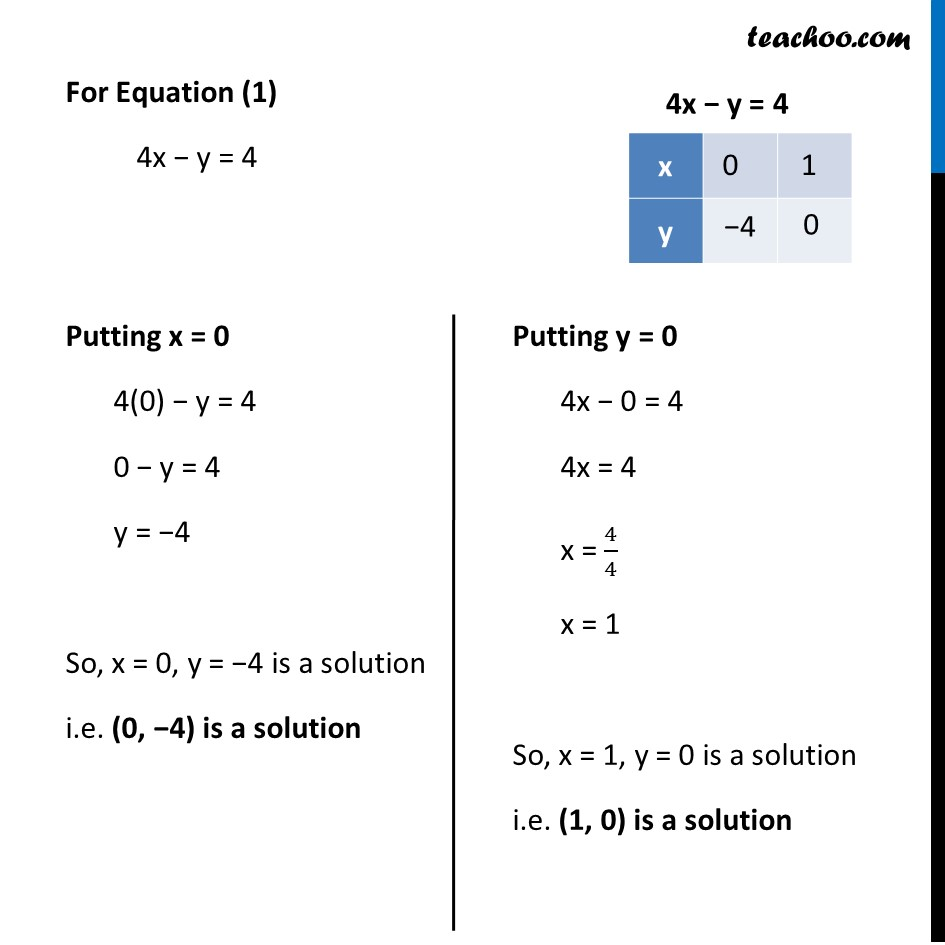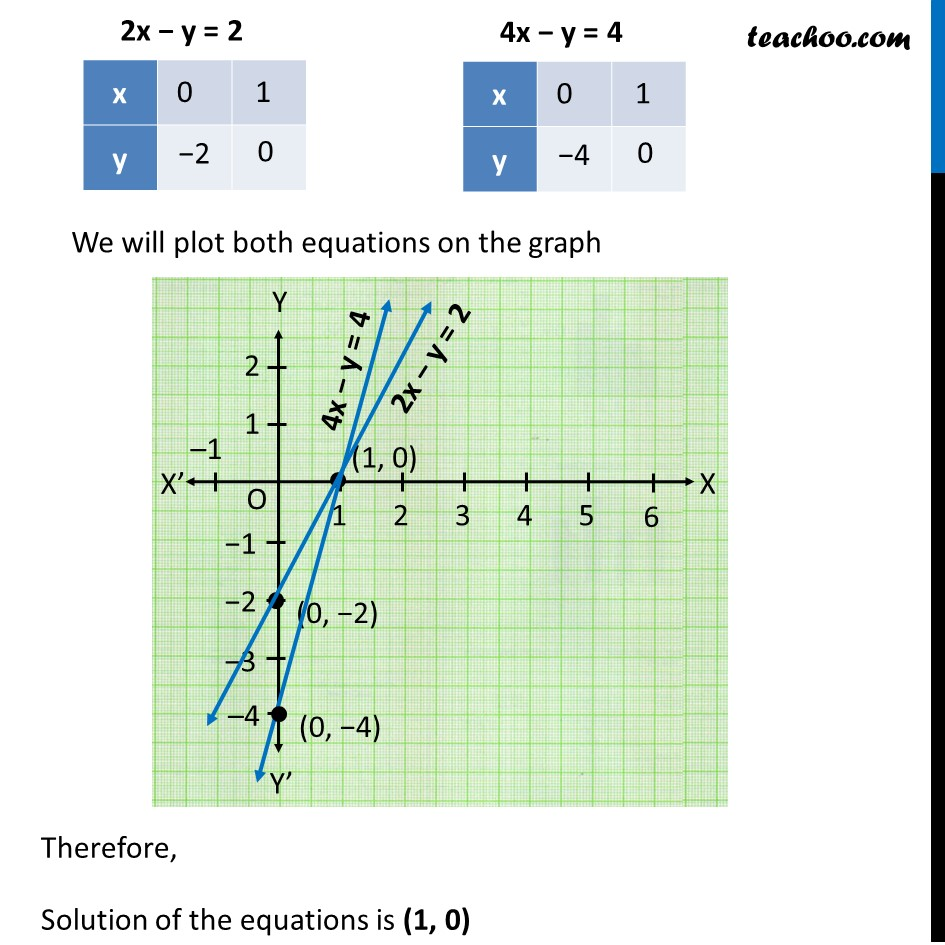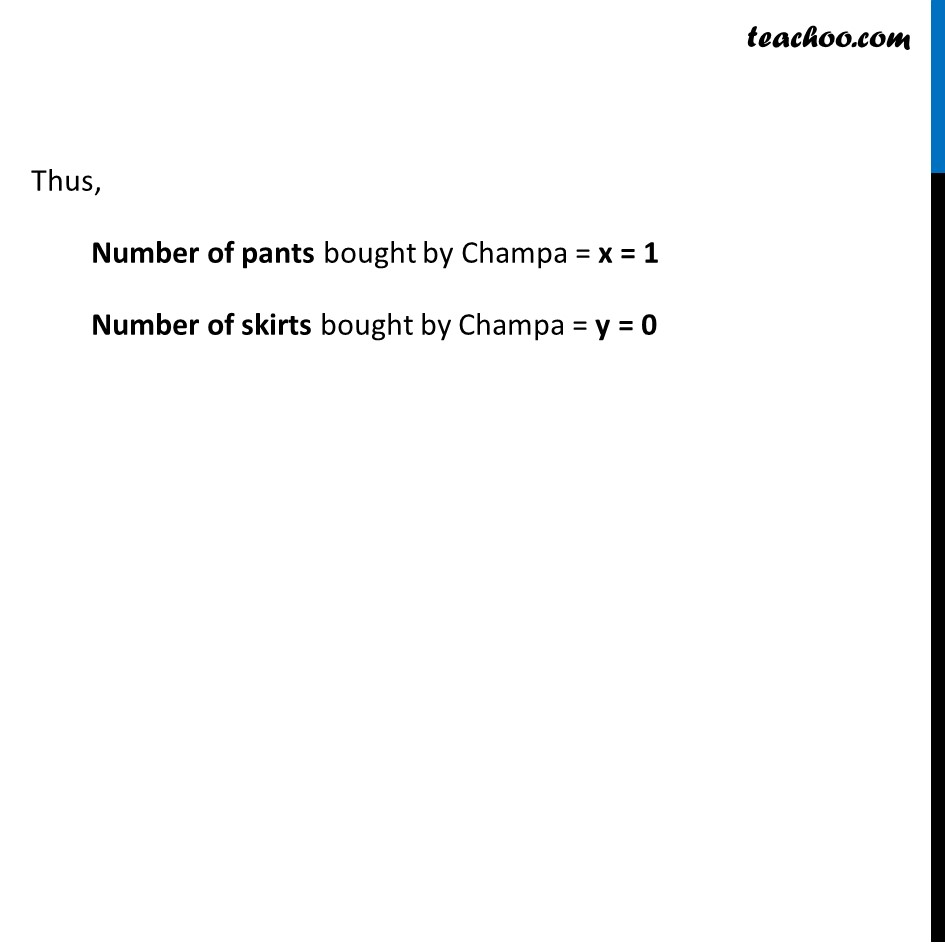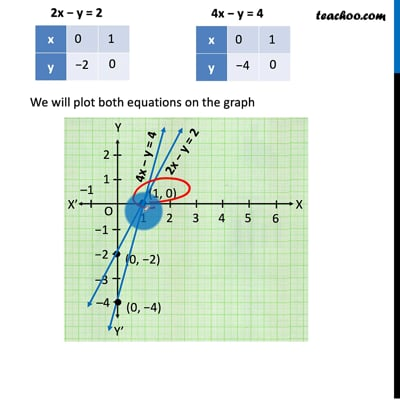This video is only available for Teachoo black users

Get live Maths 1-on-1 Classs - Class 6 to 12

### Transcript

Example 6 Champa went to a ‘Sale’ to purchase some pants and skirts. When her friends asked her how many of each she had bought, she answered, “The number of skirts is two less than twice the number of pants purchased. Also, the number of skirts is four less than four times the number of pants purchased”. Help her friends to find how many pants and skirts Champa bought. Let Number of pants purchased by Champa be x & Number of skirts purchased by Champa be y Given that Number of skirts is two less than twice the number of pants purchased (Number of skirts purchased) = 2 × (Number of pants purchased) − 2 y = 2(x) − 2 2x − y = 2 Also, Number of skirts is 4 less than 4 times the number of pants purchased (Number of skirts purchased) = 4 × (Number pants purchased) − 4 y = 4(x) − 4 4x – y = 4 Now, plotting equations 2x − y = 2 ...(1) 4x – y = 4 …(2) For Equation (1) 2x − y = 2 Putting x = 0 2(0) − y = 2 0 − y = 2 y = −2 So, x = 0, y = −2 is a solution i.e. (0, −2) is a solution Putting y = 0 2x − 0 = 2 2x = 2 x = 2/2 x = 1 So, x = 1, y = 0 is a solution i.e. (1, 0) is a solution For Equation (1) 4x − y = 4 Putting x = 0 4(0) − y = 4 0 − y = 4 y = −4 So, x = 0, y = −4 is a solution i.e. (0, −4) is a solution Putting y = 0 4x − 0 = 4 4x = 4 x = 4/4 x = 1 So, x = 1, y = 0 is a solution i.e. (1, 0) is a solution We will plot both equations on the graph Therefore, Solution of the equations is (1, 0) Thus, Number of pants bought by Champa = x = 1 Number of skirts bought by Champa = y = 0